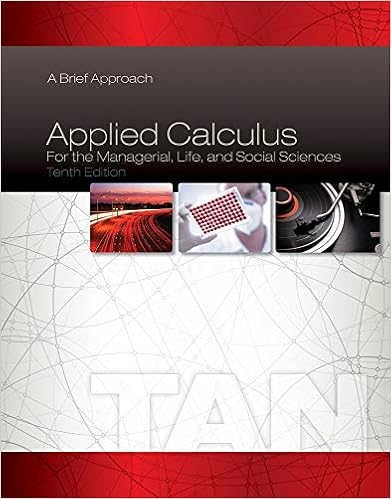In graph the function and its parent function then

• 10
• 0% (2) 0 out of 2 people found this document helpful

This preview shows page 3 - 6 out of 10 pages.

We have textbook solutions for you!
The document you are viewing contains questions related to this textbook.The document you are viewing contains questions related to this textbook.
Chapter 1 / Exercise 1
Applied Calculus for the Managerial, Life, and Social Sciences: A Brief Approach
TanExpert Verified
In Exercises 19 26, graph the function and its parent function. Then describe the transformation. (See Ex. 4.) 21. f ( x ) = 2 x 2 26. x x f 2 1 ) ( In Exercises 27 34, use a graphing calculator to graph the function and its parent function. Then describe the transformations. (See Example 5.) *33. 4 1 2 ) 3 ( ) ( x x f USING TOOLS In Exercises 39 44, identify the function family and describe the domain and range. Use a graphing calculator to verify your answer. 39. g ( x ) = | x + 2| 1 41. g ( x ) = 3 x + 4 43. f ( x ) = 5 x 2 53. REASONING Compare each function with its parent function. State whether it contains a horizontal translation, vertical translation, both, or neither . Explain your reasoning. a. 3 2 ) ( x x f b. 2 ) 8 ( ) ( x x f c. 4 2 ) ( x x f d. 2 4 ) ( x x f 2
We have textbook solutions for you!
The document you are viewing contains questions related to this textbook.The document you are viewing contains questions related to this textbook.
Chapter 1 / Exercise 1
Applied Calculus for the Managerial, Life, and Social Sciences: A Brief Approach
TanExpert Verified
Section 1.2 In Exercises 17 22, write a function g whose graph represents the indicated transformation of the graph of f. Use a graphing calculator to check your answer. (See Example 3.) 17. f ( x ) = x + 2; vertical stretch by a factor of 5 20. f ( x ) = | x + 3| ; horizontal stretch by a factor of 4 ANALYZING RELATIONSHIPS In Exercises 23 26, match the graph of the transformation of f with the correct equation shown. Explain your reasoning. A. ) ( 2 x f y B. ) 2 ( x f y C. ) 2 ( x f y D. 2 ) ( x f y Write a function g whose graph represents the indicated transformations of the graph of f . (See Example 4.) 28. x x f ) ( ; translation 3 units down followed by 31. x x f ) a vertical shrink by a factor of 3 34. Identify and correct the error in writing the function g whose graph represents the indicated transformation of ) ( x f 35. MAKING AN ARGUMENT Your friend claims that when writing a function whose graph represents a combination of transformations, the order is not important. Is your friend correct? Justify your answer. ( 1 .
CC Algebra II Name _________________________ HW #3 Period ___ Row ____ Date ______ Modeling With Linear Equations Read 1.3 Examples 1, 2, 3 Section 1.3
•••